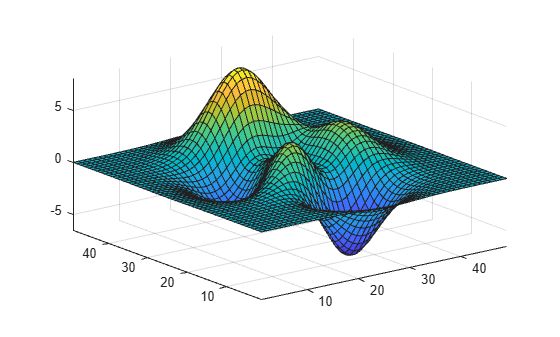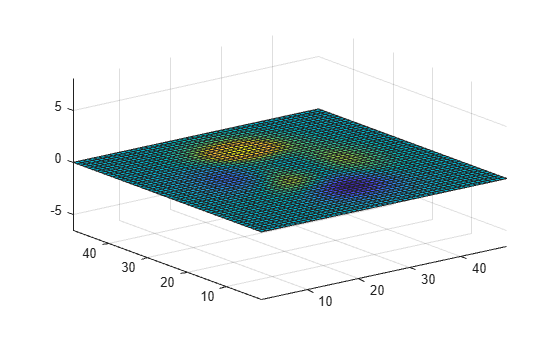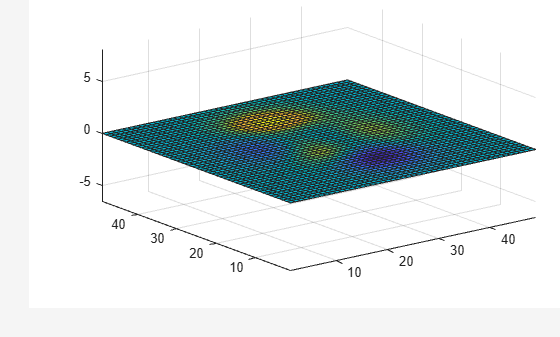# movie

Play recorded movie frames

## Syntax

``movie(M)``
``movie(M,n)``
``movie(M,n,fps)``
``movie(h,...)``
``movie(h,M,n,fps,loc)``

## Description

example

````movie(M)` plays the movie frames in array `M` once. To create a movie frame from the current figure or axes, see `getframe`. ```

example

````movie(M,n)` plays the movie `n` number of times. You can specify `n` as a numeric array, where the first element of the array specifies the number of times to play the movie, and the remaining elements make up a list of frames to play consecutively in the movie. ```

example

````movie(M,n,fps)` specifies the number of frames per second `fps` at which to play the movie.```

example

````movie(h,...)` plays the movie centered in the figure or axes identified by the figure or axes object `h`. Specifying the figure or axes enables MATLAB® to fit the movie to the available size.```

example

````movie(h,M,n,fps,loc)` plays the movie at the position specified by a four-element array `loc` in the current figure.```

## Examples

collapse all

Use the `getframe` function in a loop to record frames of the `peaks` example function, then play the movie frames once.

Create a `figure` object `h`. Initialize the surface plot of the `peaks` function `Z`. Customize the figure axes.

```h = figure; Z = peaks; surf(Z) axis tight manual ax = gca; ax.NextPlot = 'replaceChildren';```

Preallocate a 40-element array `M` to store the movie frames.

```loops = 40; M(loops) = struct('cdata',[],'colormap',[]);```

For each iteration of `j`, capture each plot of function `X` as an individual frame. Store the frame in `M`.

Set the `'Visible'` property of the figure object to `'off'` to hide the surface plots while calculating `X`.

```h.Visible = 'off'; for j = 1:loops X = sin(j*pi/10)*Z; surf(X,Z) drawnow M(j) = getframe; end```

Set the `'Visible'` property of the figure to `'on'` and play the movie in `M` once.

`h.Visible = 'on';``movie(M);`

View the figure plots while they are calculated. Play the movie array once.

Create a `figure` object `h` and initialize the surface plot. Customize the figure axes.

```h = figure; Z = peaks; surf(Z) axis tight manual``````ax = gca; ax.NextPlot = 'replaceChildren';```

Preallocate a 40-element array `M` to store the movie frames.

```loops = 40; M(loops) = struct('cdata',[],'colormap',[]);```

Capture each plot of function `X` as an individual frame and store them in `M`.

```for j = 1:loops X = sin(j*pi/10)*Z; surf(X,Z) drawnow M(j) = getframe; end```Play the movie in `M` once.

`movie(M);`

Play four frames of the movie array twice.

Create a `figure` object `h` and initialize the surface plot. Customize the figure axes.

```h = figure; Z = peaks; surf(Z) axis tight manual ax = gca; ax.NextPlot = 'replaceChildren';```

Preallocate a 40-element array `M` to store the movie frames.

```loops = 40; M(loops) = struct('cdata',[],'colormap',[]);```

Capture each plot of function `X` as an individual frame and store them in `M`.

Set the `'Visible'` property of the figure object to `'off'`.

```h.Visible = 'off'; for j = 1:loops X = sin(j*pi/10)*Z; surf(X,Z) drawnow M(j) = getframe; end```

Set the `'Visible'` property of the figure to `'on'`. Play the first, fifteenth, twenty-third, and thirty-sixth frames in the movie in consecutive order twice. Set the movie to play 12 frames per second.

`h.Visible = 'on';``movie(M,[2 1 15 23 36],12);`

Specify the number of frames per second at which to play the movie.

Create a `figure` object `h` and initialize the surface plot. Customize the figure axes.

```h = figure; Z = peaks; surf(Z) axis tight manual ax = gca; ax.NextPlot = 'replaceChildren';```

Preallocate a 40-element array `M` to store the movie frames.

```loops = 40; M(loops) = struct('cdata',[],'colormap',[]);```

Capture each plot of function `X` as an individual frame and store them in `M`.

Set the `'Visible'` property of the figure object to `'off'`.

```h.Visible = 'off'; for j = 1:loops X = sin(j*pi/10)*Z; surf(X,Z) drawnow M(j) = getframe; end```

Set the `'Visible'` property of the figure to `'on'`. Play the movie once at 6 frames per second.

`h.Visible = 'on';``movie(M,1,6);`

Play the movie frames in the bottom left corner of the figure.

Create a `figure` object `h` and initialize the surface plot. Customize the figure axes.

```h = figure; Z = peaks; surf(Z) axis tight manual ax = gca; ax.NextPlot = 'replaceChildren';```

Preallocate a 40-element array `M` to store the movie frames.

```loops = 40; M(loops) = struct('cdata',[],'colormap',[]);```

Set the `'Visible'` property of the figure object to `'off'`. Capture each figure in handle `h` as an individual frame and store them in `M`.

```h.Visible = 'off'; for j = 1:loops X = sin(j*pi/10)*Z; surf(X,Z) drawnow M(j) = getframe(h); end```

Set the `'Visible'` property of the figure to `'on'`. Play the movie in `M` once at 12 frames per second and with an offset of 30 pixels in the x and y directions. Specify handle `h` to play the movie in the current figure.

`h.Visible = 'on';``movie(h,M,1,12,[30 30 0 0]);`

## Input Arguments

collapse all

Array of movie frames, specified as an array of structures, where each structure contains the image data captured in the current figure or axes as they appear on the screen. Each row in `M` corresponds to one movie frame.

You can use the `getframe` function to capture and store the image data as a structure with the fields `cdata` and `colormap`. For more information on the `cdata` image property and the `colormap` object, see Image Properties and `colormap`.

Number of times to play movie in the figure, specified as a numeric array. If you do not specify `n`, `movie` will only play the movie once. If `n` is negative, each cycle is shown forward then backward.

If you specify `n` as a vector, the first element is the number of times to play the movie, and the remaining elements make up a list of frames to play in the movie.

For example, if `M` consists of four movie frames and `n` is an array with elements `n = [10 4 4 2 1]`, then `movie` plays the movie in `M` ten times, and the movie consists of the fourth frame, followed by the fourth frame again, followed by the second frame, and finally the first frame.

Frames per second, specified as a numeric scalar. If you do not specify `fps`, `movie` will play 12 frames per second. If your machine cannot achieve the speed that you specify as `fps`, it will play the movie as fast as possible.

Graphics object handle, specified as an axes handle or a figure handle. The default handle of `h` is `gca`, which returns the current axes or chart for the current figure. For more information on the `gca` function, see `gca`.

If you want to play the movie in the figure instead of the axes, specify the figure handle (or `gcf`) as the first argument: `movie(figure_handle,...)`.

Location vector, specified as a four-element numeric array, ```[x y 0 0]```, where the lower left corner of the movie frame is anchored at the coordinates specified by the first two elements in the array. The coordinates specified in `loc` are in relation to the lower left corner of the figure or axes specified by handle h, and are in units of pixels. The `movie` function ignores the last two elements of `loc`.

## Tips

• The `movie` function uses a default figure size of 560-by-420 and does not resize figures to fit movies with larger or smaller frames. To accommodate other frame sizes, you can resize the figure to fit the movie.

• Buffering the movie places all frames in memory. As a result, on Microsoft® Windows® and perhaps other platforms, a long movie (on the order of several hundred frames) can exhaust memory, depending on system resources. In such cases an error message is issued:

```??? Error using ==> movie Could not create movie frame```

You can abort a movie by typing Ctrl-C.